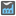• # My wrong code passes the test

## Question related to missionNumbers Factory

My code returns 34 for `checkio(12)`. Of course, a correct solution should return 26. I believe a test should be added to check how the users code handles prioritizing 2s and 3s. There are other bugs in my code too that would be caught by testing products of powers of 2s and 3s.

For reference, here is my inelegant, incorrect code:

```def prime_factors(number):
""" Returns a list of the prime factors of number, includes multiplicity"""
factors = []
for p in range(2, number+1):
while number % p == 0:
factors.append(p)
number /= p
if p > number: break
return factors

def checkio(number):
factors = prime_factors(number)
if any(p > 10 for p in factors):
return 0
else:
while factors.count(2) > 2:
# Replace three 2s with 8
factors = factors[3:] + 
if factors == 2 or factors == 3:
# Replace two 2s or a 2 and a 3 with 4 or 6, respectively
factors = factors[2:] + [factors * factors]
while factors.count(3) > 1:
# Replace two 3s with a 9
factors = factors[2:] + 

return int("".join(str(p) for p in sorted(factors)))
```14